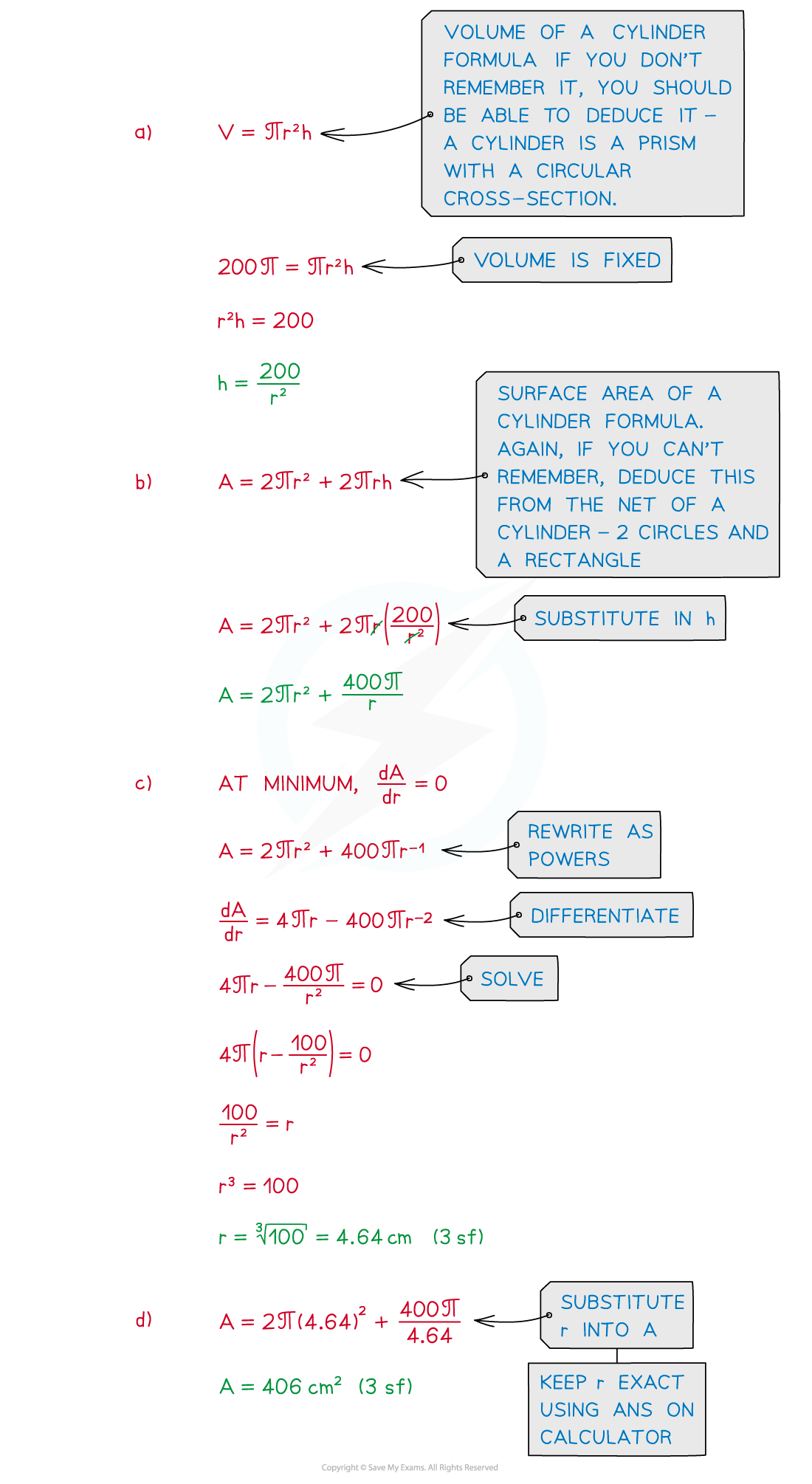# Edexcel IGCSE Maths 复习笔记 3.11.3 Differentiation - Problem Solving

Edexcel IGCSE Maths 复习笔记 3.11.3 Differentiation - Problem Solving

#### What problems could involve differentiation?

• Differentiation allows analysis of how one quantity changes as another does
• The gradient function (derivative) gives a measure of the rate of change
• Problems involving a variable quantity can involve differentiation
• How the area of a rectangle changes as its length varies
• How the volume of a cylinder changes as its radius varies
• How the position of a car changes over time (ie. speed – see Kinematics)

• Problems based on the graph of a curve may also arise
• The distance between two turning points
• The area of a shape formed by points on the curve such as turning points and axes intercepts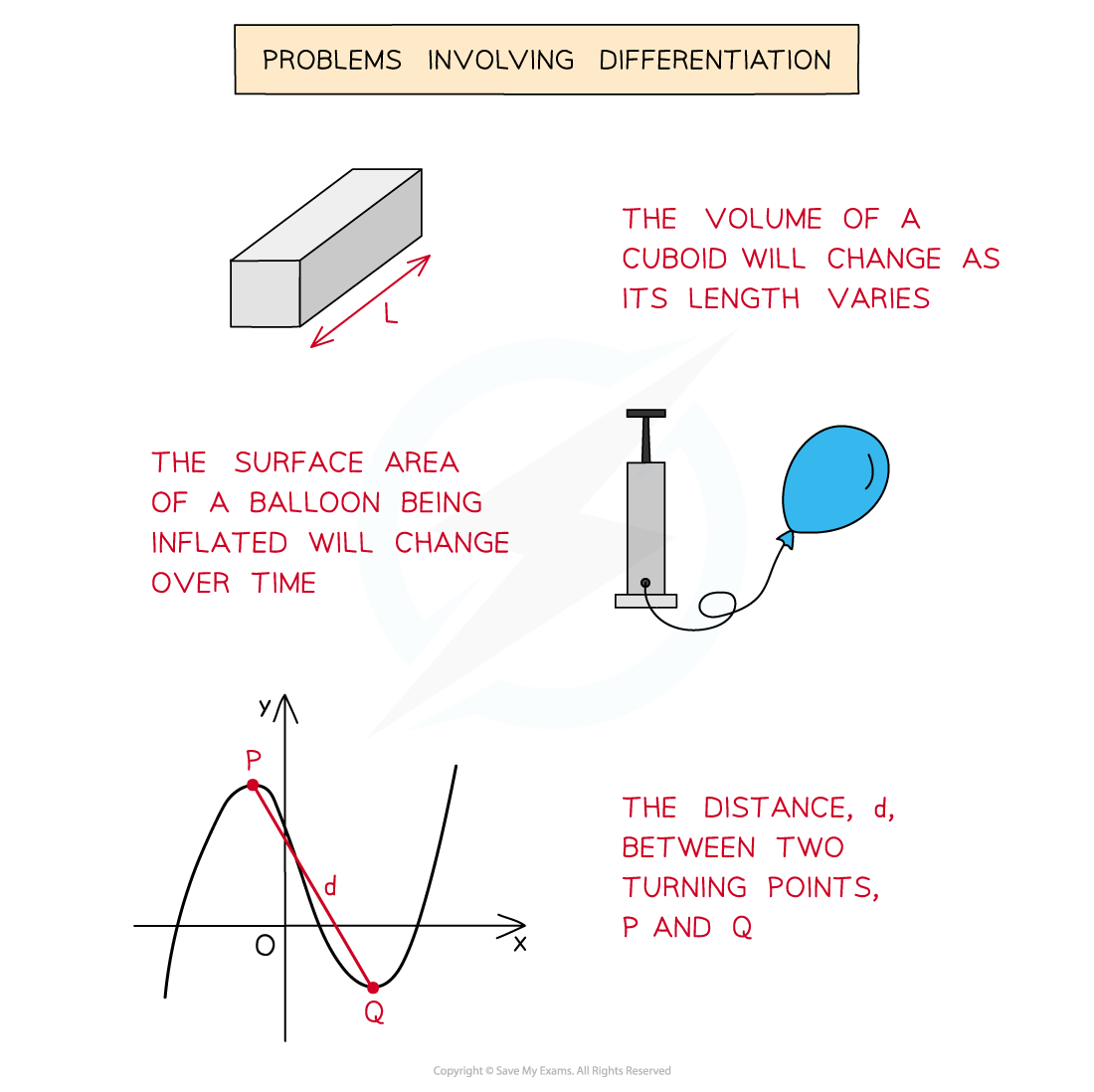#### How do I solve problems involving differentiation?

• Problems generally fall into two categories

1. Graph based problems

• These problems are based around the graph of a curve and its turning points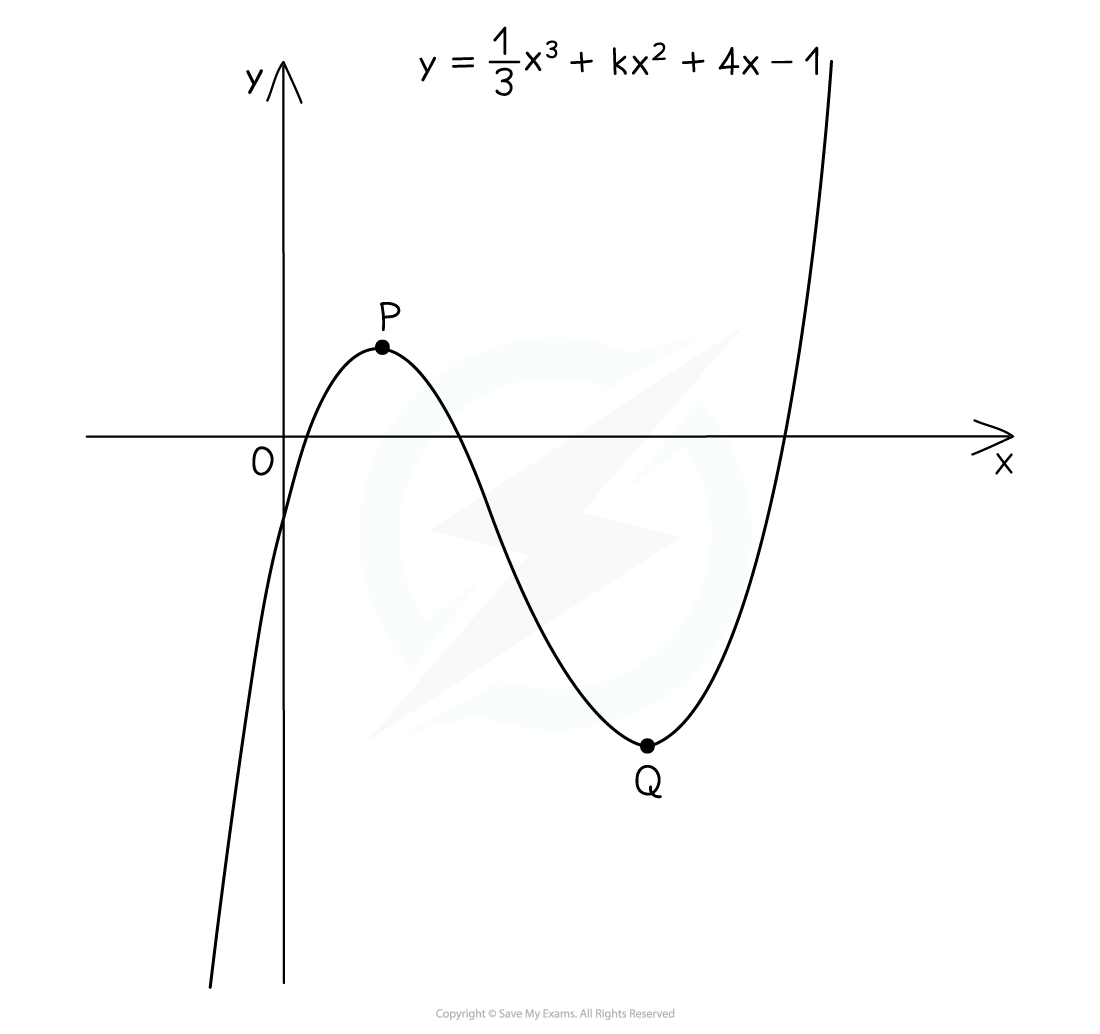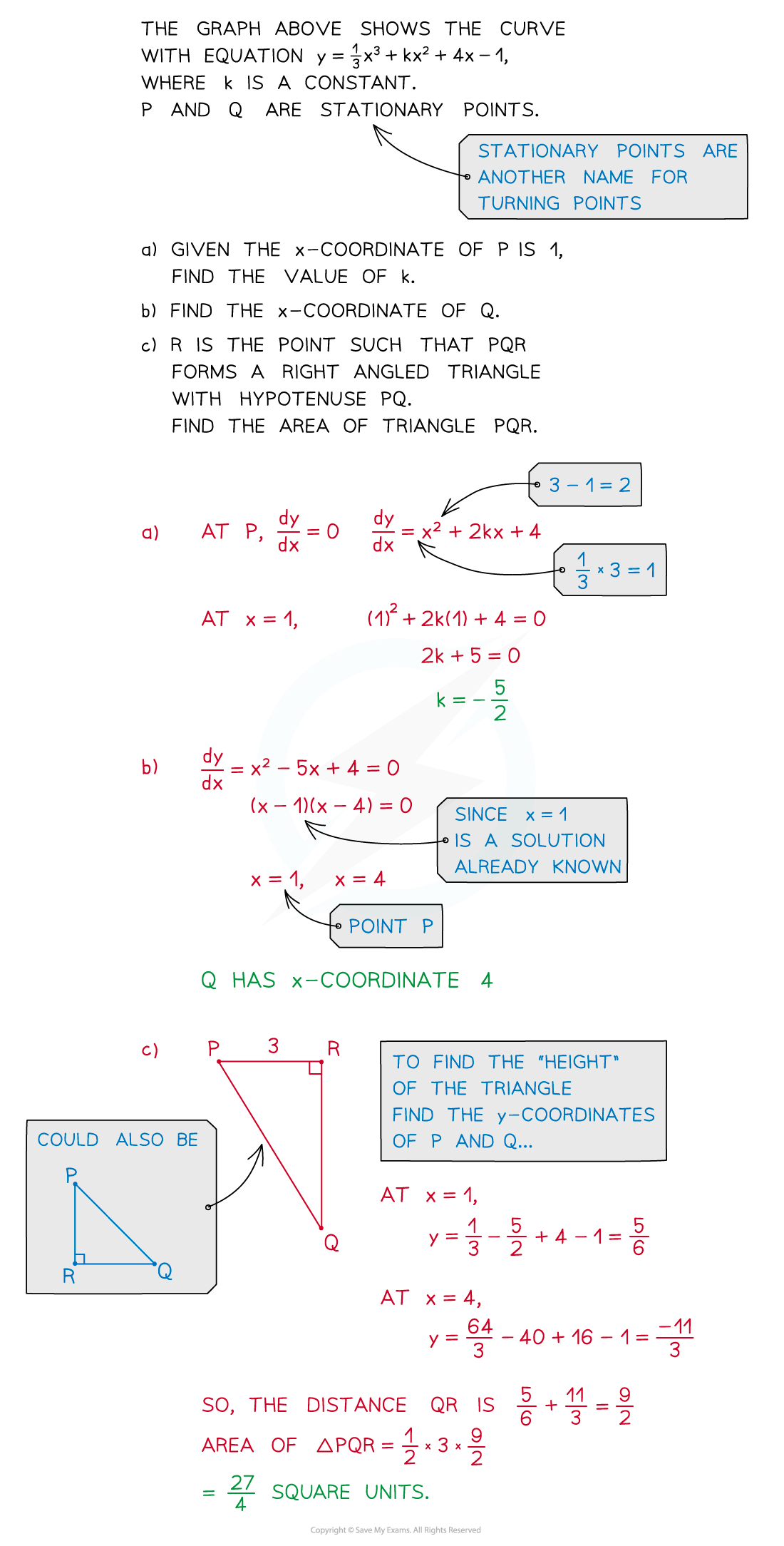2. Maximum/Minimum problems

• The maximum or minimum values have a meaning in the question
• eg the maximum volume of a box made from a flat sheet of material
• eg the minimum height of water in a reservoir

• These are sometimes called optimisation problems
• The maximum or minimum value gives the optimal (ideal/best)solution to the problem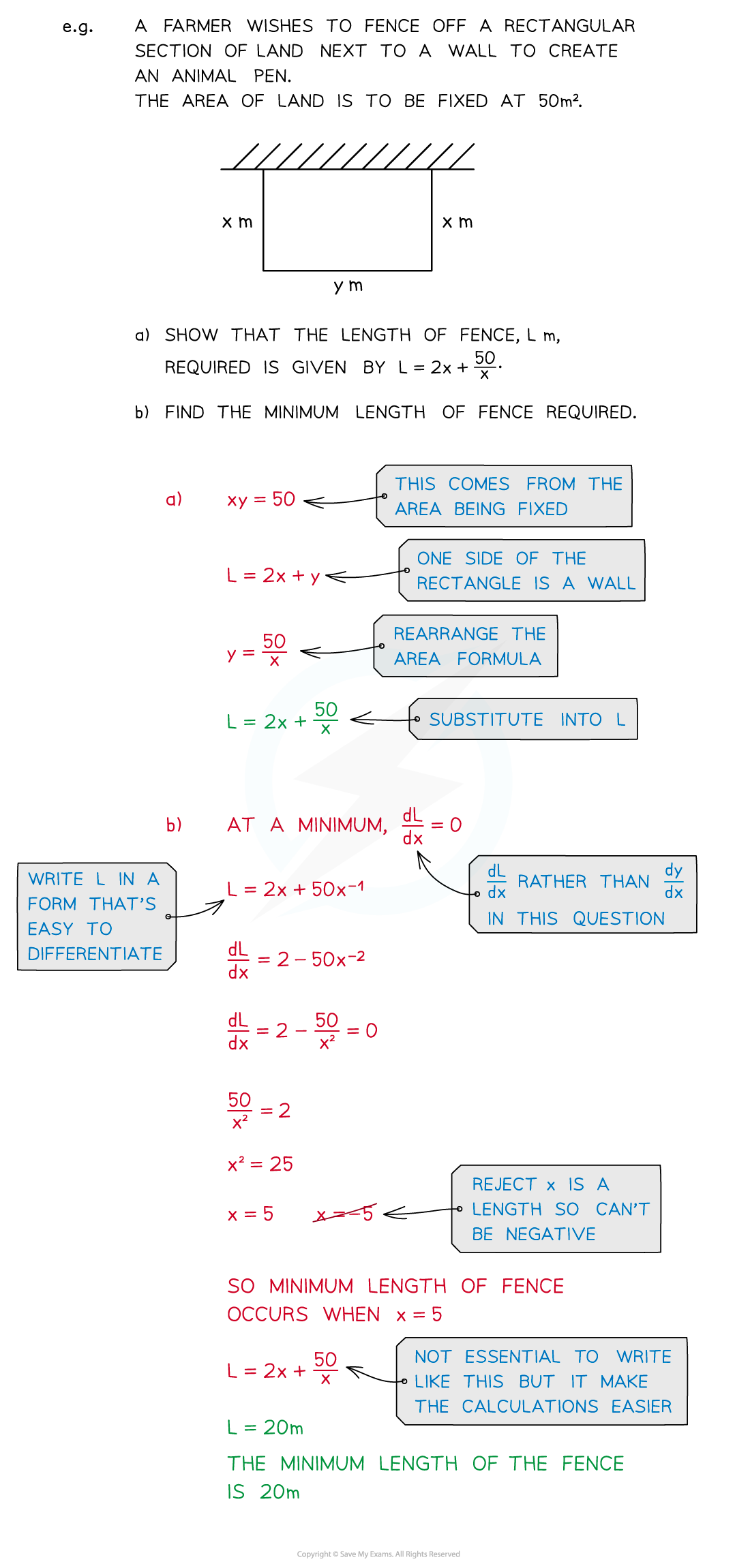• A third type of problem involving the motion of an object is dealt under the separate set of notes Kinematics

#### Exam Tip

Diagrams can help – if you are not given one, draw one – a sketch is usually good enough; add to the diagram as you progress through the question.Make sure you know how to find the areas and volumes of basic shapes eg. area of squares, rectangles, triangles, circles, volume of cubes, cuboids, cylindersEarly parts of questions often ask you to “show that” a result is true.

Even if you can’t do this part of the question, the result may still be used in other parts allowing you to score some marks.

#### Worked Example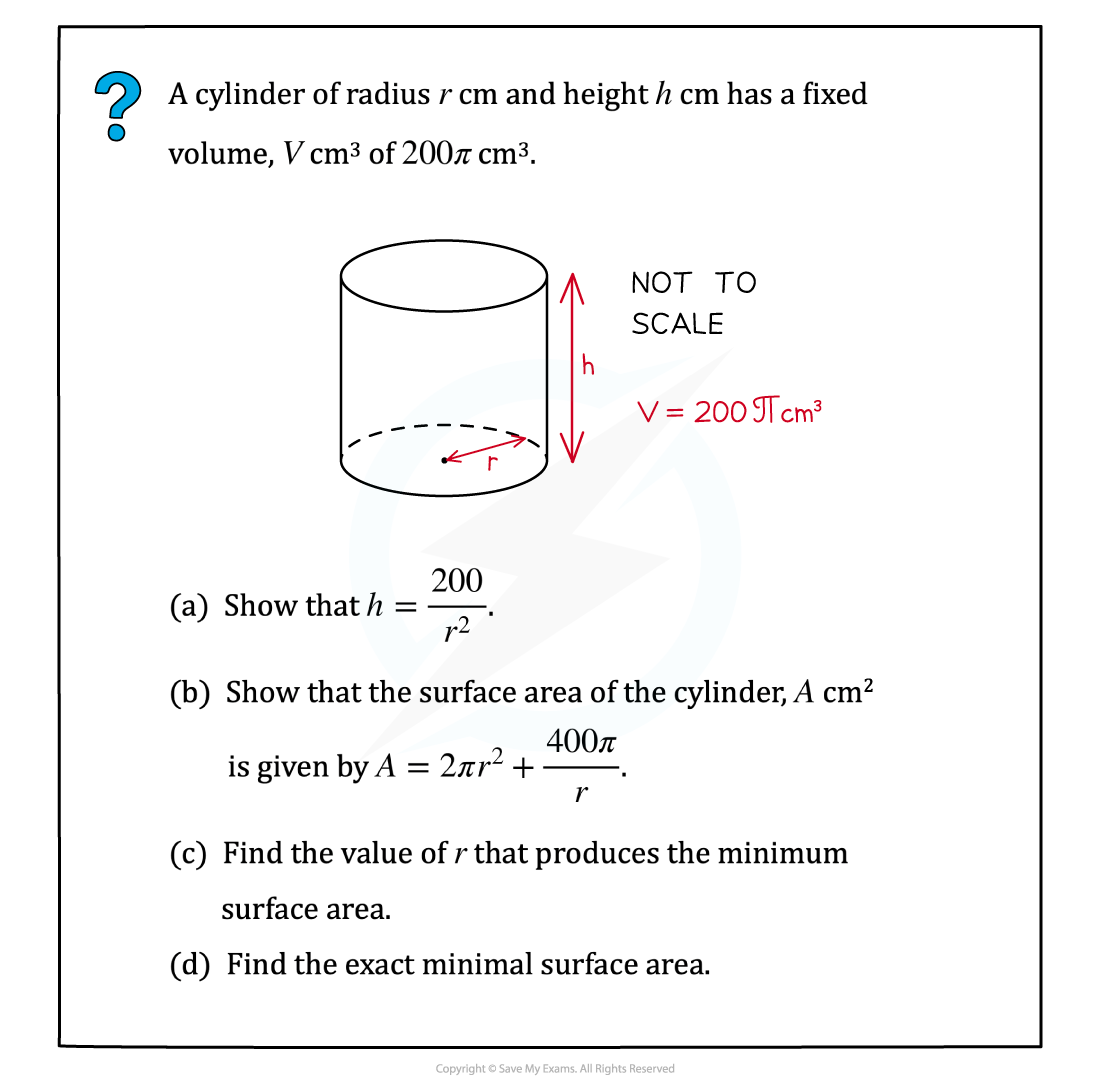#### Worked Example